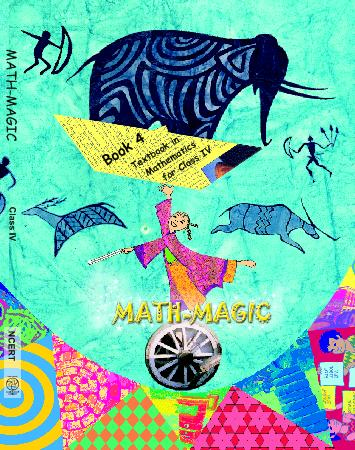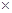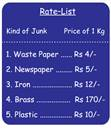1. /
2. CBSE
3. /
4. Class 04
5. /
6. Mathematics
7. /
8. NCERT Solutions for Class...

# NCERT Solutions for Class 4 Maths The Junk Seller

NCERT solutions for Class 4 Mathematics## NCERT Solutions for Class 4 Maths The Junk Seller

Chapter -6 Class 4 Maths The Junk Seller

### 1. What about you? Do you also find Maths difficult?

Ans. I do not find Maths difficult.

### 2. What is the most difficult thing in you Maths book?

Ans. The chapter entitled “The Way the World Looks” is the most difficult thing in Maths book.

### 3. What do you think is the easiest lesson?

Ans. The lesson “A Trip to Bhopal” is the essential.

### 4. Find out: how much for a cup of tea?

Ask people and find out the cost of a cup of tea

(a) At a tea stall

Ans. (a) At a tea stall, the cost of a cup is Rs 3.

(b) At a hotel

Ans. (b) At a hotel, the cost of a cup is Rs 10.

### 5. If a person who runs a tea stalls earns Rs 30 in a day, how much will he earn in 10 days? And in a month? How did you get the answer? Discuss.

Ans. Earning of a tea stall owner per day = Rs 30

Therefore, earning of a tea stall owner in 10 days = Rs (1030) = Rs 300

And his, earning in a month = Rs (3030) = Rs 900

I got the answers by multiplication.

### 6. Find out: What is a loan?

(a) Have you ever heard of someone taking a loan? For what?

Ans. (a) Yes, I have heard of someone taking a loan. It is taken to buy a handcart.

(b) How much loan was taken?

Ans. (b) Rs 300 was taken as loan.

(c) How much money was paid back.

Hariya and Babu went to buy a handcart for Rs 300.

Ans. (c) Hariya: I have taken a loan of Rs 300 from a Bank for six months. I will pay Rs 51 every month to the bank.

Babu: But I have taken a loan of Rs 300 from Chunnilal. After six months, I will pay back Rs 360.

(d) Who has to pay back more- Hariya or Babu?

Ans. (d) In case of Hariya: Money paid back to the bank = Rs. 651 = Rs 306

In case of Babu: Money paid back to Chunnilal = Rs 360

Since, Rs 360 is more than Rs 300, therefore, Babu paid back more money.

### 7. (a) Have 9 rickshaws of my own. I give the rickshaws on rent, each of Rs 20 a day. On Sundays I do not take any money from them? How much does Kiran earn from 9 Rickshaws in a Day?

Ans. (a) For 1 rickshaw she gets Rs 20 per day.

So, for 9 rickshaws she will earn Rs 180.

(b) How did you do it?

Ans. (b) I did it by multiplying 20 and 9 (209=180).

### 8. In a week how much does Kiran earn from one rickshaw?

Ans. Since on Sundays Kiran does not take any money for rickshaw’s rent, therefore, Kiran earns in a week on one rickshaw at the rate of Rs 20 per day = Rs 620 = Rs 120.

### 9. Do it mentally and write the answers:

(a) 26= __

Ans. (a) 26= 12.

(b) 206 = __

Ans. (b) 206 = 120.

(c) 260 = __

Ans. (c) 260 = 120.

(d) 342 = __

Ans. (d) 342 = 126.

(e) 480 = __

Ans. (e) 480 = 320.

(f) 481 = __

Ans. (f) 481 = 324.

(g) 925 = __

Ans. (g) 925 = 225.

(h) 319 = __

Ans. (h) 319 = 279.

Ans. Yes.

### 11. How much Kiran will pay for 31 kg newspaper?

Ans. Kiran will pay for 31 kg newspaper = Rs (5130 + 51) = Rs 155.

12.(a) How much Kiran pay for 42 kg newspaper?

Ans. (a) Kiran will pay Rs (425) = Rs 210.

(b) Also find the costs of:

(i) 22 kg of plastic

Ans. (b) (i) Cost of 22 kg of plastic = Rs (2210) = Rs 220.

(ii) 23 kg of wastepaper

Ans. (b) (ii) Cost of 23 kg of newspaper = Rs (234) = Rs 92.

(iii) 12 kg of iron.

Ans. (b) (iii) Cost of 12 kg of iron = Rs (1212) = Rs 144.

### 13. Can you do this without writing? Guess the total money Kiran will pay to the junk collectors. Will it be: More than 600? Less than 600?

Ans. Less than 600.

### 14. Kiran bought 1 kg of plastic for Rs 10, but sold 1 kg plastic for Rs 12. How much money does she earn on selling 1 kg plastic?

Ans. Earning on selling 1 kg of plastic = Selling price – Cost price = Rs 12 – Rs 10 = Rs 2

So, earning on selling 63 kg of plastic = Rs 2Rs 63

 60 3 602 32 120 6

On adding the numbers in the last row = 120 + 6 = 126.

So, for 63 kg of plastic, Kiran earns = Rs 126.

Class 4 Maths The Junk Seller

### 15. How much money will Dinu pay for 32 kg iron?

Ans. The rate of 1 kg of iron is Rs 14. So, the cost for 32kg of iron is Rs 1432.

We write as:

 30 2 10 3010 210 300 20 4 304 24 120 8

So, for 32 kg iron, Dinu will pay Rs 448.

### 16. Kiran buys 1 kg iron for Rs 12, but sells it for Rs 14. How much does she earn when she sells 32 kg iron?

Ans. Earning of selling 1 kg iron = Selling price -Cost price = Rs 14 – Rs 12 = Rs 2

So, earning on selling 32 kg of iron = Rs 232 of iron = Rs 232

We write as:

 30 2 302 22 60 4

So, Kiran earns Rs 64 on the sale of 32 kg iron.

Ans. Rs 152.

### 18. How much money will Dinue pay for 4 kg brass? Guess the answer first.

Ans. The rate of 1 kg brass is Rs 170. So, the cost of 4 kg brass is Rs 1704. We guess the answer to lie between Rs 600 and Rs 800.

We write as:

 4 100 70 1004 704 400 280

So, for 4 kg brass Dinu will pay Kiran Rs 680.

### 19. First guess the answer then calculate:

(a) 3718

Ans. (a) On guessing 3718 may be = 740.

We write as:

 30 7 10 3010 710 300 70 8 308 78 240 56

Adding the numbers = 300 + 240 + 70 + 56 = 666

So, 3718 = 666.

### (b) 4524

Ans. (b) On guessing 4524 = 1080

We write as:

 40 7 20 4020 520 800 100 4 404 54 160 20

Adding the numbers = 800 + 160 + 100 + 20 = 1080

So, 4524 = 1080

(c) 6952

Ans. (c) On guessing 6952 = 3588

We write as:

 60 9 50 6050 950 3000 450 2 602 92 120 18

Adding the numbers = 3000 + 120 + 450 + 18 = 3588

So, 6952 = 3588.

(d) 7755

Ans. (d) On guessing 7755 = 4235

We write as:

 70 7 50 7050 750 3500 350 5 705 75 350 35

Adding the numbers = 3500 + 350 + 350 + 35 = 4235

So, 7755 = 4235.

(e) 1425

Ans. (e) On guessing 1425 = 710

We write as:

 100 40 2 5 1005 405 25 500 200 10

Adding the numbers = 500 + 200 + 10 = 710

So, 1425= 710.

(f) 3823

Ans. (f) On guessing 3823 =1146

We write as:

 300 80 2 3 3003 803 23 900 240 6

Adding the numbers = 900 + 240 + 6 = 1146

So, 3823 = 1146

(g) 2175

Ans. (g) On guessing 2175 = 350

We write as:

 100 70 5 2 1002 702 52 200 140 10

Adding the numbers = 200 + 140 + 10 = 350

So, 2175 = 350.

(h) 4206

Ans. (h) On guessing 4206 = 824

We write as:

 200 0 6 4 2004 04 64 800 0 24

Adding the numbers = 800 + 0 + 24 = 824

So, 4206 = 824.

## NCERT solutions for Class 4 Mathematics Chapter 6 The Junk Seller

Building with BricksView Solutions
Long and ShortView Solutions
A trip to BhopalView Solutions
Tick Tick TickView Solutions
The Way the World LooksView Solutions
The Junk SellerView Solutions
Jugs and MugsView Solutions
Carts and WheelsView Solutions
Halves and QuartersView Solutions
Play With PatternsView Solutions
Table and SharesView Solutions
How Heavy How LightView Solutions
Fields And FencesView Solutions
Smart ChartsView Solutions

NCERT Solutions Class 4 Maths Class 4 Maths The Junk Seller PDF (Download) Free from myCBSEguide app and myCBSEguide website. Ncert solution class 4 Maths includes text book solutions from Class 4 Maths Book . NCERT Solutions for CBSE Class  Maths have total 14 chapters. 4 Maths NCERT Solutions in PDF for free Download on our website. Ncert Maths class 4 solutions PDF and Maths ncert class 4 PDF solutions with latest modifications and as per the latest CBSE syllabus are only available in myCBSEguide.

## CBSE app for Students

To download NCERT Solutions for Class 4 Maths, EVS Hindi English, Maths Science do check myCBSEguide app or website. myCBSEguide provides sample papers with solution, test papers for chapter-wise practice, NCERT solutions, NCERT Exemplar solutions, quick revision notes for ready reference, CBSE guess papers and CBSE important question papers. Sample Paper all are made available through the best app for CBSE students and myCBSEguide website.### Test Generator

Create question paper PDF and online tests with your own name & logo in minutes.### myCBSEguide

Question Bank, Mock Tests, Exam Papers, NCERT Solutions, Sample Papers, Notes